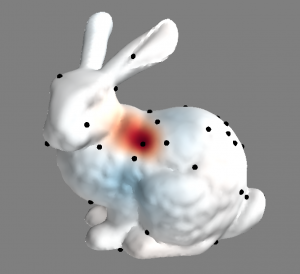## A New Method to Approximate Symmetric Matrices

Symmetric matrices are very common in many fields. For example, they are used in Kernel Machines to  maintain pairwise kernel functions, while in computer vision they represent pairwise distances between points. When a dataset contains ten thousands or more points, these symmetric matrices do not fit in memory and may be too expensive to compute. Existing alternatives suggest to approximate this matrix using a low-rank approximation. Nyström is a very powerful method that samples a subset of the data points and uses them to approximate the matrix. Many research has centered around theory, sampling schemes, and accuracy improvements. However, the method suffers from some practical limitations: it may become unstable when the matrix is not positive semidefinite, and when applied to millions of data points the memory requirements growth, limiting the accuracy of the method.

With Professor Alex Huth, we developed a new method to approximate symmetric matrices that overcomes these limitations. We dubbed our method biharmonic matrix approximation (BHA). Assuming the data points reside in a manifold, BHA samples a subset of data points and interpolates the manifold from this subset using Biharmonic interpolation. The method computes the symmetric matrix of this sampled subset and utilizes the interpolation to approximate the results to the other points. This means of approximation avoids numerical instabilities that exists in other methods. Moreover, the interpolation construction of BHA assigns higher weights to nearby sampled point than to those farther away. From this observation, we construct a sparse version of BHA that reduces the memory consumption enabling approximation of millions of data points and similar accuracy.The figure depicts the weights for the black vertex in the red zone. The weights are over all the other vertices on the mesh. Red symbolizes positive values, blue negative values and white a near-zero value. This weights represent a column of the interpolation operator of BHA.

## Learning a Functional Alignment Model with a Semi-Supervised Approach

A few days ago, our paper “A Semi-supervised Method for Multi-Subject fMRI Functional Alignment” was accepted to the IEEE International Conference on Acoustics, Speech and Signal Processing that will be held next March in New Orleans, Louisiana, USA. This work presents an extension to the original Shared Response Model (SRM), an unsupervised method for multi-subject functional alignment of fMRI data. Using a semi-supervised approach, we show how to train SRM taking into consideration data from a supervised task (multi-label classification). In this way, we need almost half the number of unlabeled samples to achieve the same accuracy level, or achieve higher accuracy with the same number of unlabeled samples.

The method extends the deterministic SRM formulation with a Multinomial Logistic Regression penalty. The semi-supervised SRM inherits the characteristics of the SRM problem, defining a non-convex optimization problem. We solve it using a block-coordinate descent approach, where each block is an unknown matrix. We show similarities to the SRM and MLR, and note that finding the mappings requires to solve an optimization problem in the Stiefel manifold. While this has an closed-form in the SRM case, in the SS-SRM this requires general techniques to solve it. We use the excellent pymanopt package that allowed us to implement a solution for python. Also, the source code of SS-SRM has been published as part of the Brain Imaging Analysis Kit (BrainIAK).